Question

Questions 1. Calculate the molarity of a sodium hydroxide (NaOH) solution that is titrated with 0.6887...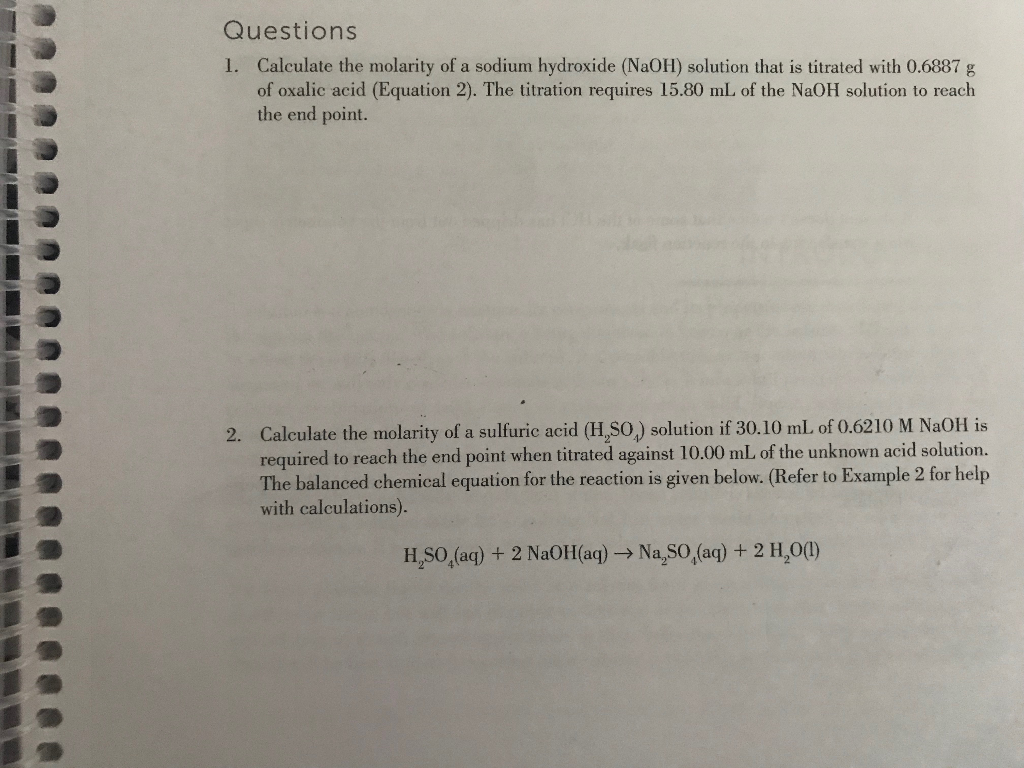Questions 1. Calculate the molarity of a sodium hydroxide (NaOH) solution that is titrated with 0.6887 g of oxalic acid (Equation 2). The titration requires 15.80 mL of the NaOH solution to reach the end point. Calculate the molarity of a sulfuric acid (H,SO) solution if 30.10 mL of 0.62 10 M NaOH is required to reach the end point when titrated against 10.00 mL of the unknown acid solution. The balanced chemical equation for the reaction is given below. (Refer to Example 2 for help with calculations) 2. Na,SO,(aq) + 2 H,00) H,SO,(aq) +2 NaOH(aq) -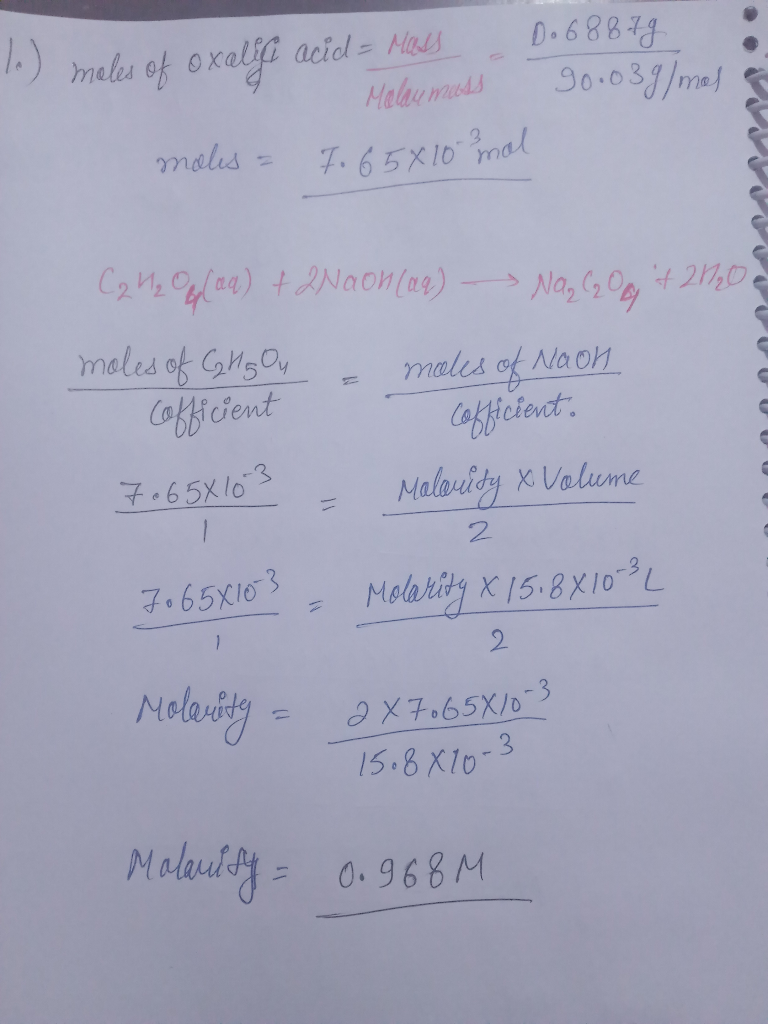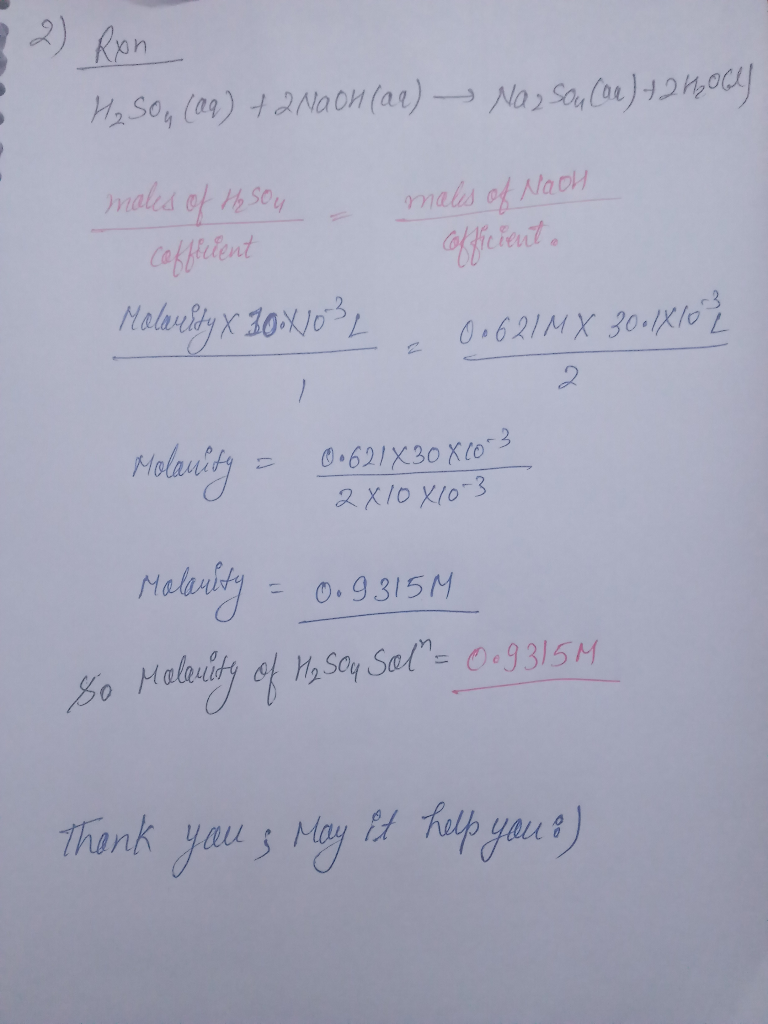Earn Coins

Coins can be redeemed for fabulous gifts.

Similar Homework Help Questions
• Titration and Molarity Problems 1. What is the molarity of a sodium hydroxide solution if 9...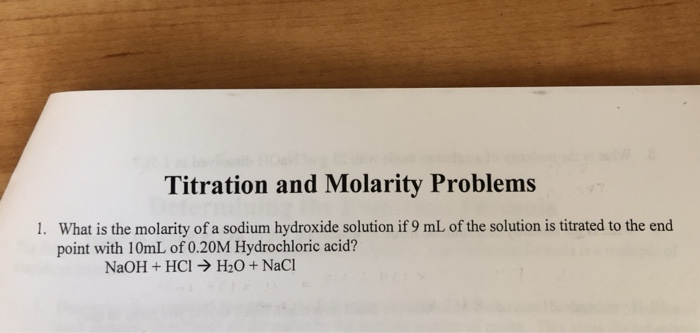Titration and Molarity Problems 1. What is the molarity of a sodium hydroxide solution if 9 mL of the solution is titrated to the end point with 10mL of 0.20M Hydrochloric acid? NaOH + HCl → H2O + NaCl

• Standardization of a Sodium Hydroxide Solution Section Name Score Date Post-lab Assignment 1. Calculate the molarity...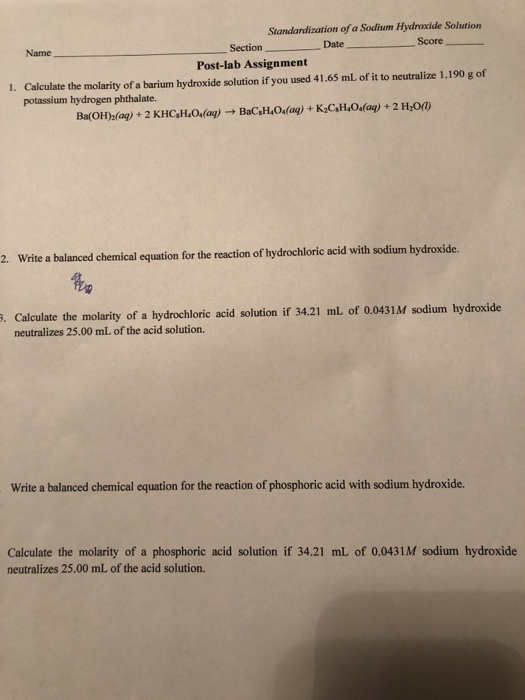Standardization of a Sodium Hydroxide Solution Section Name Score Date Post-lab Assignment 1. Calculate the molarity of a barium hydroxide solution if you used 41.65 mL of it to neutralize 1.190 g of potassium hydrogen phthalate. Ba(OH)2(aq) + 2 KHC4H6O4(aq) + BaC,H,O4(aq) + K2CH4O4(aq) + 2 H300) 2. Write a balanced chemical equation for the reaction of hydrochloric acid with sodium hydroxide. 3. Calculate the molarity of a hydrochloric acid solution if 34.21 mL of 0.0431M sodium hydroxide neutralizes 25.00...

• A sulfuric acid solution of 20.0 mL is titrated with NaOH of 0.100 molarity. The solution...

A sulfuric acid solution of 20.0 mL is titrated with NaOH of 0.100 molarity. The solution with phenolphthalein turns pink when 32.16 mL of NaOH is added. Every acidic hydrogen is neutralized. Find the grams, molarity, and normality of sulfuric acid before the titration begins.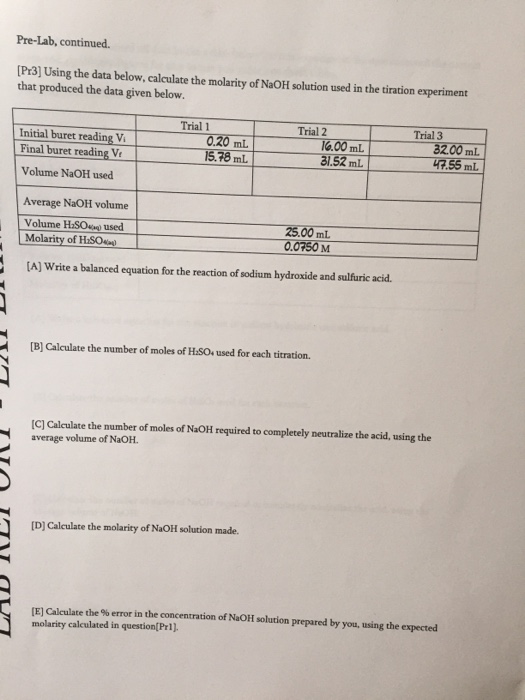Please help me to answer... Using the data below, calculate the molarity of NaOH solution used in the titration experiment that produced the data given below. Write a balanced equation for the reaction of sodium hydroxide and sulfuric acid. Calculate the number of moles of H_2SO_4 used for each titration. Calculate the number of moles of NaOH required to completely neutralize the acid, using the average volume of NaOH. Calculate the molarity ofNaOH solution made. Calculate the % error in...

• 10.00 mL Nitric acid was titrated with NaOH of 0.100 molarity until the equivalence was reached. 15.2 mL of NaOH were re...

10.00 mL Nitric acid was titrated with NaOH of 0.100 molarity until the equivalence was reached. 15.2 mL of NaOH were required. Find: A) Concentration of nitric acid before titration B) pH of solution before titration C) pH of solution after 15.1 mL of sodium hydroxide is added D) pH of solution after 15.3 mL of sodium hydroxide is added

• What is the molarity of the sodium hydroxide solution used in the titration? 0.4750 M In...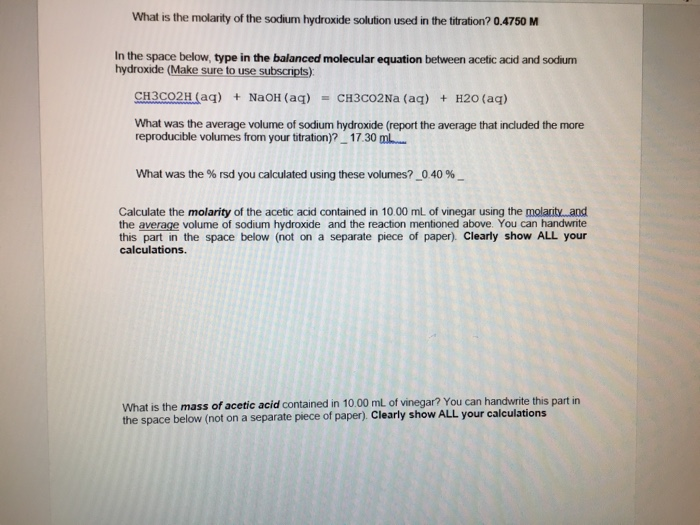What is the molarity of the sodium hydroxide solution used in the titration? 0.4750 M In the space below, type in the balanced molecular equation between acetic acid and sodium hydroxide (Make sure to use subscripts) CH3CO2H (aq) NaOH (aq)CH3CO2Na (aq) H20 (aq) What was the average volume of sodium hydroxide (report the average that included the more reproducible volumes from your ttration)? 17.30 m. What was the % rsd you calculated using these volumes?,040 %- Calculate the molarity of...

• 50.00 mL of unknown calcium hydroxide solution is titrated with 0.300 M standard nitric acid solution....

50.00 mL of unknown calcium hydroxide solution is titrated with 0.300 M standard nitric acid solution. If 42.21 mL of the standard acid so lution is required to reach a phenolphthalein endpoint, what is the molarity of the unknown calcium hydroxide solution? (Hint: write the balanced formula unit equation.)

• Lab Titration of Acids and Bases 5. Sodium carbonate is a reagent that may be used...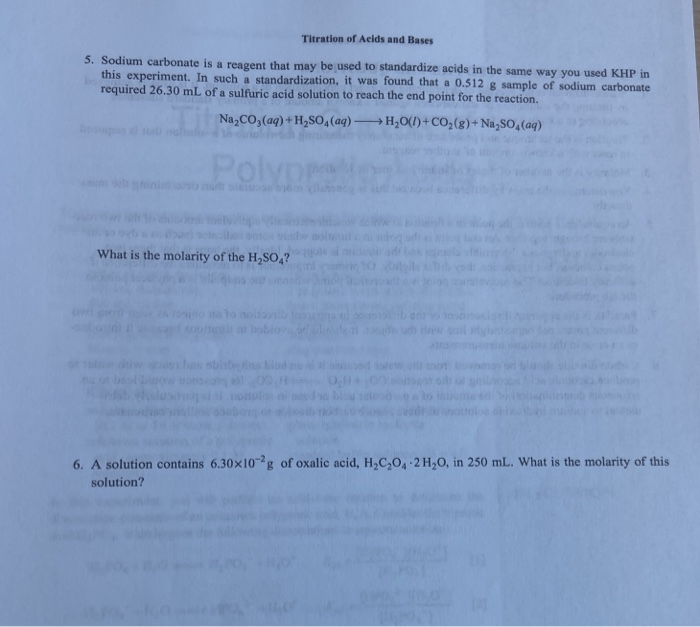Lab Titration of Acids and Bases 5. Sodium carbonate is a reagent that may be used to standardize acids in the same way you used KHP in this experiment. In such a standardization, it was found that a 0.512 sample of sodium carbonate required 26.30 mL of a sulfuric acid solution to reach the end point for the reaction. Na CO2(aq) + H2SO (aq) →H2O(1)+ CO2(8)+ Na 50 (aq) What is the molarity of the H,SO 2 6. A solution...

• B standarization of sodium hydroxide solution B. STANDARDIZATION OF SODIUM HYDRoXIDE SOLUTION (Show Work) Balanced chemical equation : Heaat2NaOHiNadach + 2H z O Trial 3 Trial 1 Trial 2 Mass of oxa...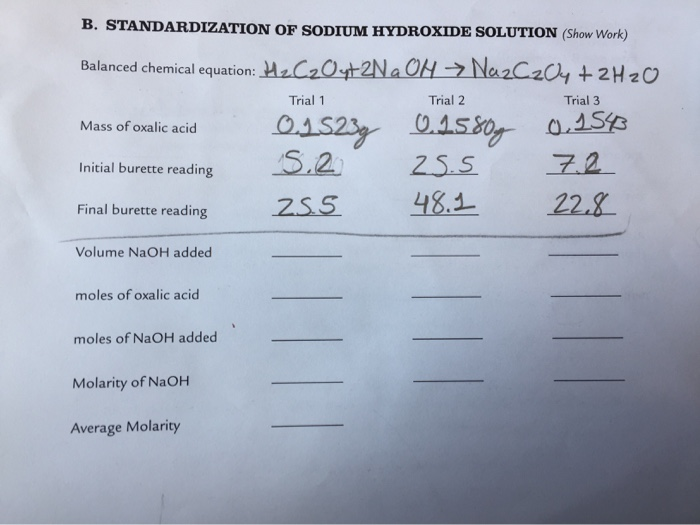B standarization of sodium hydroxide solution B. STANDARDIZATION OF SODIUM HYDRoXIDE SOLUTION (Show Work) Balanced chemical equation : Heaat2NaOHiNadach + 2H z O Trial 3 Trial 1 Trial 2 Mass of oxalic acid initial burett reading 25.S 72 Final burete reading 2SS 41 22. Volume NaOH added moles of oxalic acid moles of NaOH added Molarity of NaOH Average Molarity B. STANDARDIZATION OF SODIUM HYDRoXIDE SOLUTION (Show Work) Balanced chemical equation : Heaat2NaOHiNadach + 2H z O Trial 3 Trial...

• Weak Acid-Strong Base Titration Date Nanse PRE-LAB OUESTIONS 1 Calculate the molarity of a NaOH solution...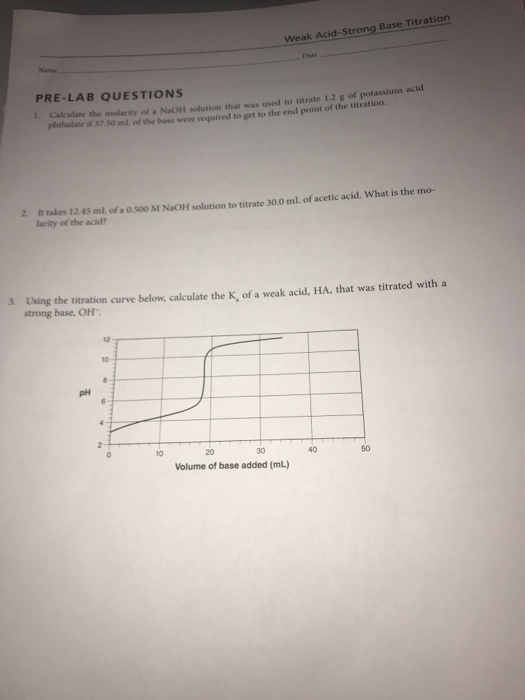Weak Acid-Strong Base Titration Date Nanse PRE-LAB OUESTIONS 1 Calculate the molarity of a NaOH solution that was used to titrate 1.2 g of potassium acid phthalate if 37.50 ml of the base were reauired to get to the end point of the titration. 2 It takes 12.45 ml. of a 0.500 M NaOH solution larity of the acid to titrate 30.0 mL of acetic acid. What is the mo- 3. Using the titration curve below, calculate the K, of...